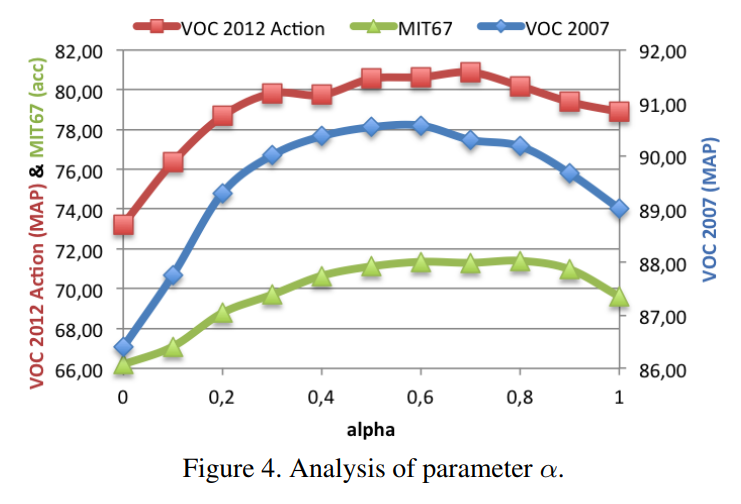## Summary

WILDCAT is a model that performs three visual recognition tasks in a weakly supervised setting:

• classification
• localization
• semantic segmentation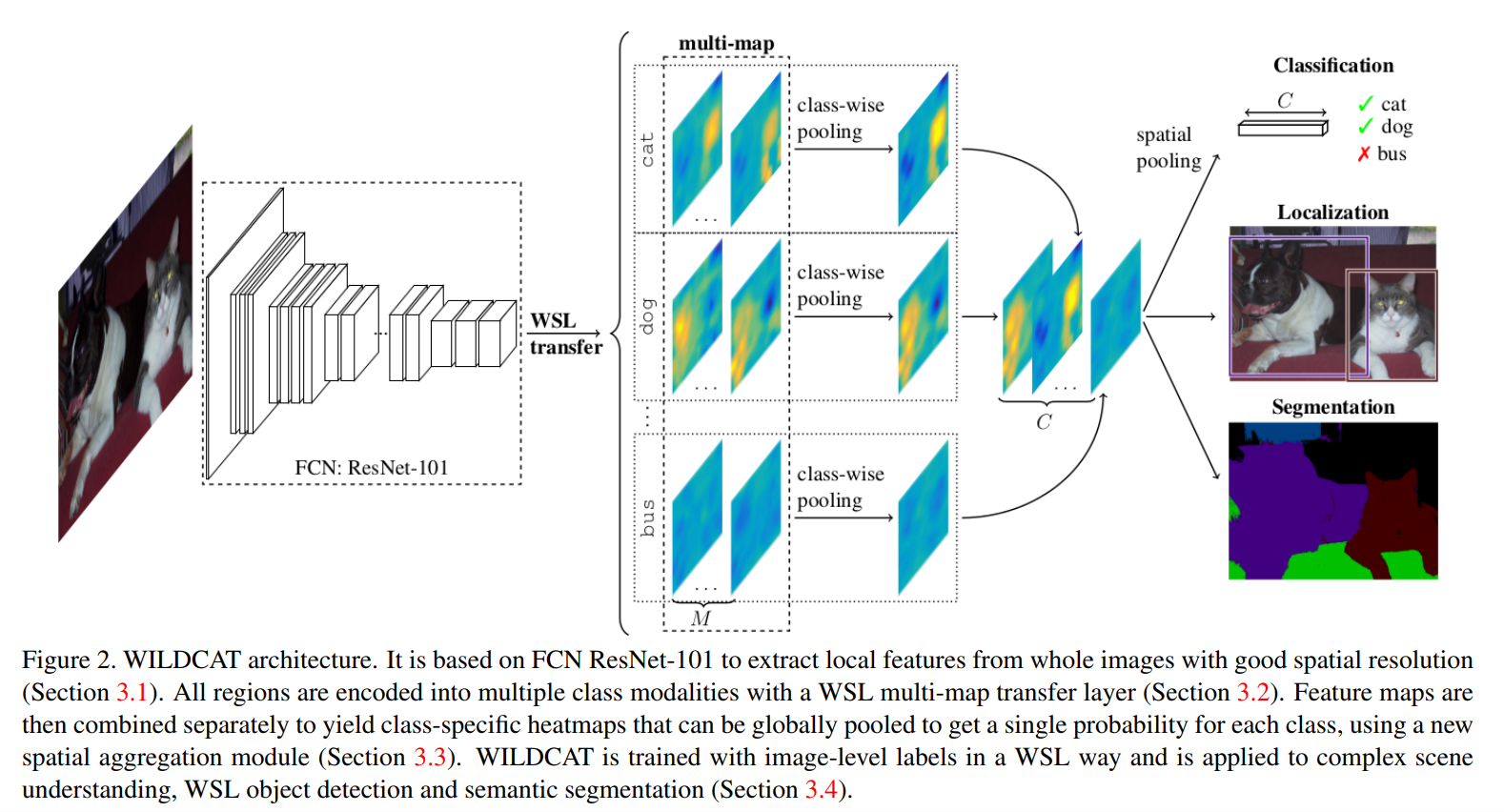The model is trained using only global image labels with the goal of aligning image regions for spatial invariance and learning strongly localized features.

A new pooling method is proposed which uses local features explicitly designed for different class modalities.

## Multi-map WSL (Weakly Supervised Learning) transfer layer

Explicitly learn multiple localized features related to class modalities (e.g. head and legs for dogs in Figure 1).

The WSL layer learns $$M$$ feature maps per class through 1x1 convolutions. These modalities are learned in a weakly supervised setup using only the image-level labels and keeping spatial resolution.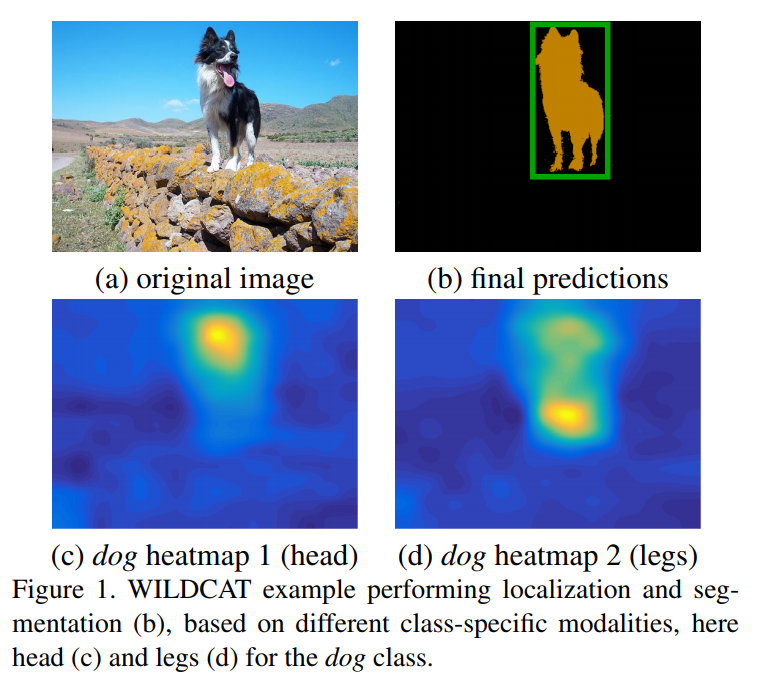## Wildcat pooling

In order to learn only from image labels, the wildcat pooling layer summarizes all information contained in the feature maps for each class.

The pooling is done in two steps:

1. a class-wise pooling combines the $$M$$ feature maps from the WSL layer (in this case, average pooling is used).
2. a spatial pooling selects relevant regions within the maps to support predictions.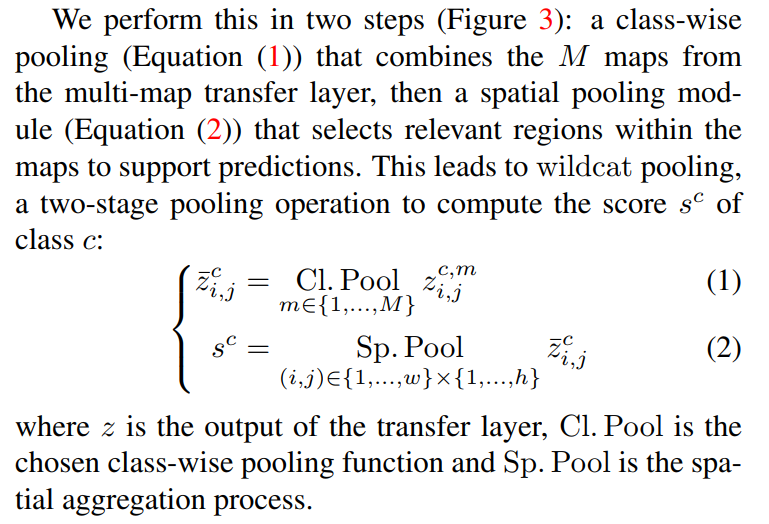Class-wise pooling

“We note that even if a multi-map followed by an average pooling is functionally equivalent to a single convolution (i.e. M = 1), the explicit structure it brings with M modalities has important practical advantages making training easier. We empirically show that M > 1 yields better results than regular M = 1”

Spatial pooling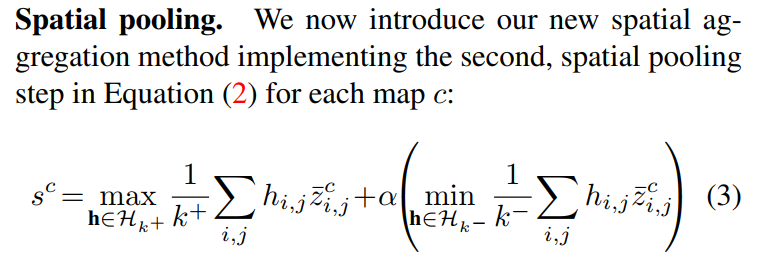h is a binary matrix with a zero-norm contraint ($$\sum_{i,j} h_{i,j} = k$$)

It corresponds to selecting the $$k^{+}$$ (resp. $$k^{-}$$) regions with the highest (resp. lowest) activations from the input $$\bar{z}^c$$.

The output of this layer for each class is the weighted average of scores for all the selected regions.

When learning WILDCAT, the gradients are backpropagated through the wildcat layer only within the $$k+$$ + $$k−$$ selected regions, all other gradients being discarded .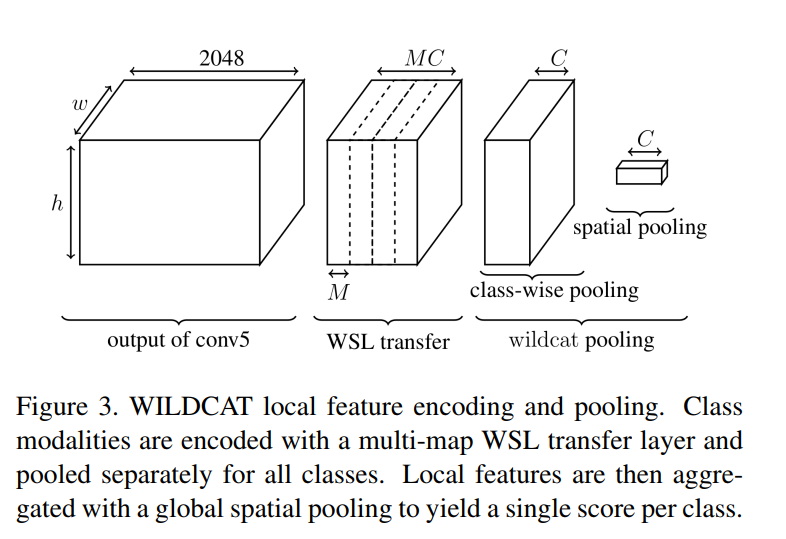## Predictions

For object detection and semantic segmentation, class-specific maps are extracted before the spatial pooling layer to keep spatial resolution.

Pointwise object detection: The region (i.e. neuron in the feature map) with maximum score for each class is extracted and used for localization.

Segmentation: Either take the class with maximum score at each spatial position or use a CRF for spatial prediction.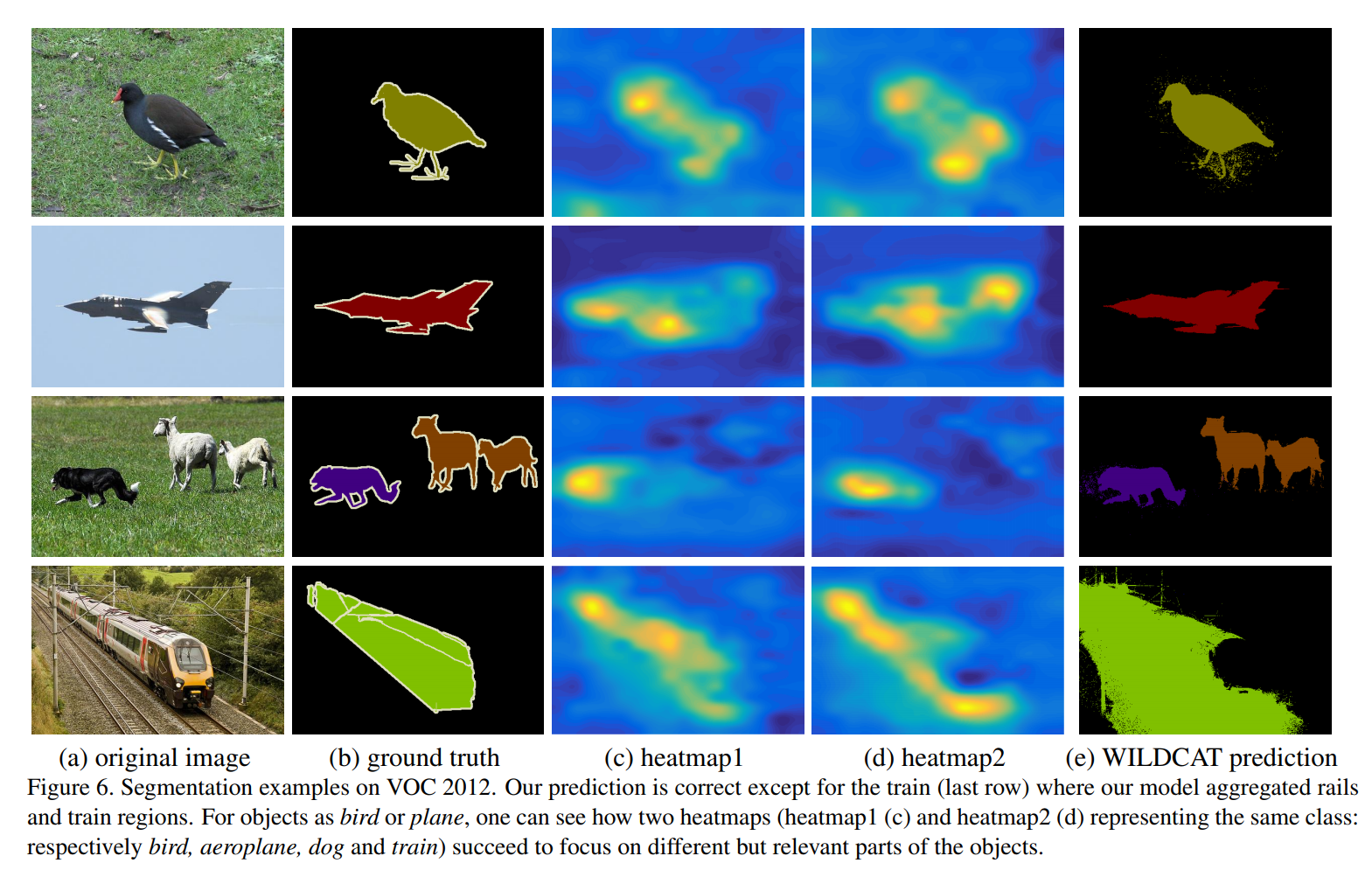## Experiments and Results

Datasets:

• Object recognition: VOC 2007, VOC2012
• Scene categorization: MIT67, 15 Scene
• Visual recognition where context is important: MS COCO, VOC 2012 Action

### Classification performance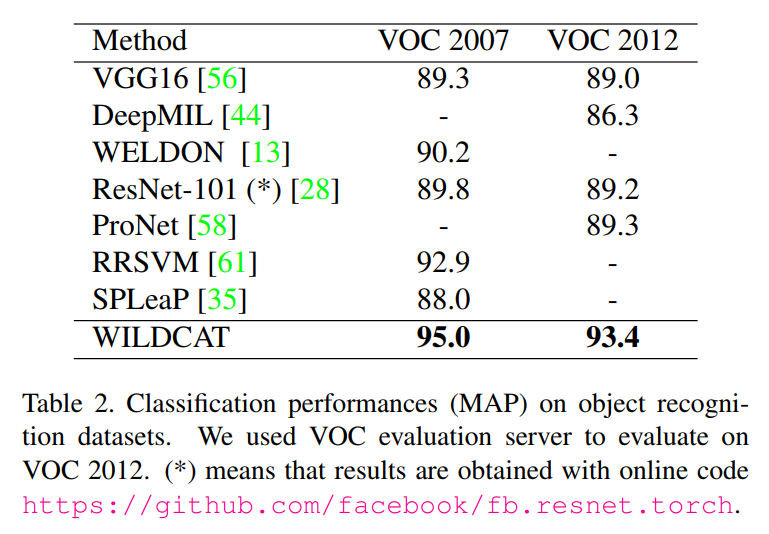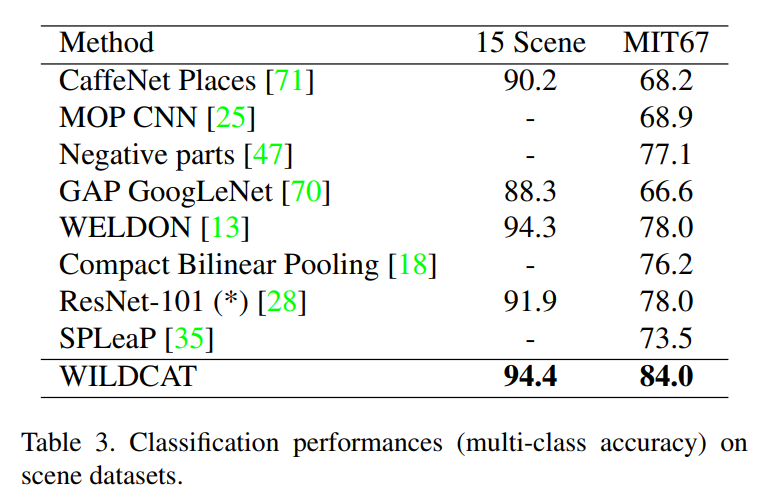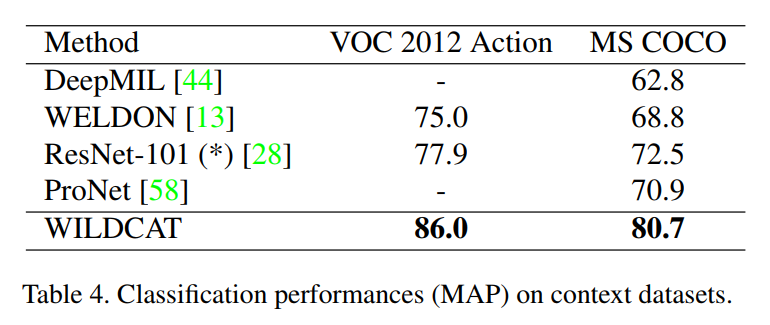### Localization performance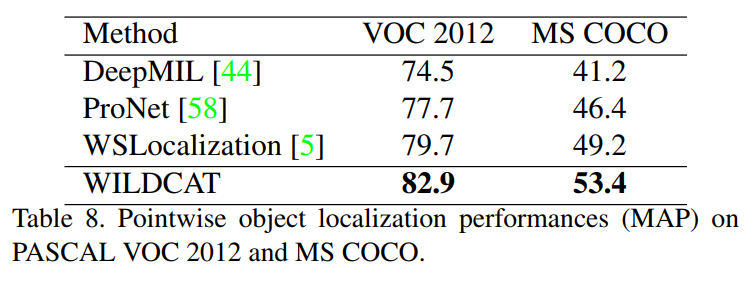### Segmentation performance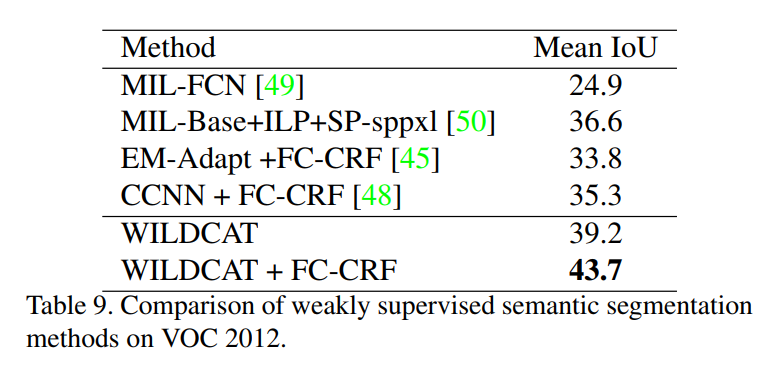### Analysis of parameter alpha